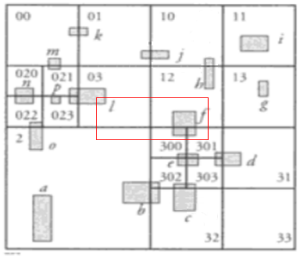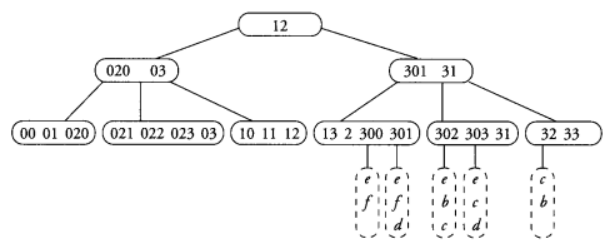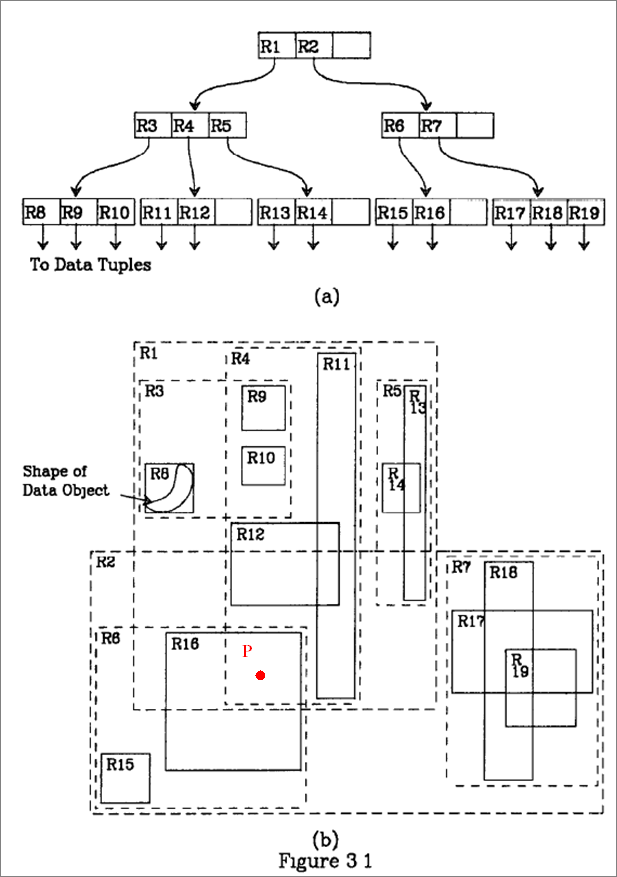﻿

## Homework 8 (due 6/2) CSC 543

We finished talking about computational geometry and saw material on spatial indexing: z-ordering trees, (linear) quadtrees, and R-trees. Most of the material was from the textbook, but I also used some other sources, including Guttman's paper introducing the R-tree and Beckmann, Kriegel, Schneider, Seeger's article introducing the R*-tree

Submission: you can submit the homework by hardcopy in class or by sending it to me as an email.

1. [Index Comparison, 15pt] Imagine you are inserting a large number of random (uniformly distributed) rectangles into a spatial index which is based on (a) a dynamic grid file, (b) a linear quadtree, (c) an R-tree

(i) For which index do you expect point queries to be fastest, for which to be slowest. Why?

(ii) For which index do you expect window queries (i.e. rectangle queries) to be fastest, for which to be slowest. Why?

2. [Linear Quadtree, 25pt] The following example shows a linear quadtree for an arrangement of rectangles (the example is taken from the textbook, we also saw it on the slides):a) Complete the linear quadtree (i.e. add the page information to the leaves: which rectangles are contained in each node?)

b) Perform a window search for the red rectangle (i.e. which rectangles intersect it); spell out the details of how the window search proceeds on the linear quadtree, which pages get accessed and how the output gets determined.

3. [R-tree, 10pt] We have seen several heuristics for splitting a node in an R-tree if, after insertion of a new rectangle, it contains too many (that is, M+1) rectangles. What about splitting the rectangles randomly, i.e. for each rectangle in turn assign it to node 1 or node 2 randomly (unless one of the nodes is full, in which you assign the rectangle to the other node)? What's the asymptotic running time of this method? Do you expect it to work well or badly? Argue your answer.

4. [R-tree, 15pt] The picture below is from Guttman's paper introducing R-trees. Perform a point query for P, i.e. find all rectangles being stored in the R-tree containing the point (to explain: R1 contains P, but is not listed, since it's a directory rectangle, not one of the rectangles stored at the leaves).  Give the details of how the algorithm proceeds through the tree, which pages get loaded, and which rectangles get checked and output.Marcus Schaefer
Last updated: May 21st, 2009.